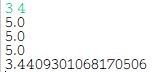## Java实验一—设计一个名为MyPoint的类_「已注销」的博客-程序员秘密

（仅记录自己的学习之路）

(1) 一个创建点（0,0）的无参构造方法；
(2) 以指定坐标构建点的构造方法；
(3) 成员变量x和y的get和set方法；
(4) 一个名为distance的静态方法，返回两个MyPoint对象之间的距离。

• 方法头：public static double distance(MyPoint p1, MyPoint p2)
• 调用Math类的sqrt方法计算平方根，方法定义如下： public static double sqrt(double a)
//返回正确舍入的 double 值的正平方根。

(5) 一个名为distance的方法，返回从该点到MyPoint类型的指定点之间的距离；

• 方法头： public double distance(MyPoint p)
• 调用上面的静态distance方法计算该点和指定点之间的距离

(6) 一个名为distance的方法，返回从该点到指定x和y坐标的另一个指定点之间的距离；

• 方法头：public double distance(double x, double y)
• 调用上面的静态distance方法计算该点和指定点之间的距离

(1) 所有成员变量用private修饰，所有方法用public修饰，画出该类的UML图并实现这个类；
(2) 编写一个测试程序，分别测试3个distance方法，将测试程序和MyPoint类放在不同的包里；

MyPoint类代码如下：

``````package MyPoint;

public class MyPoint {

private double x;
private double y;
public MyPoint() {

this.x = 0;
this.y = 0;
}                                      //创建点(0,0)的无参构造方法
public MyPoint(double x, double y) {

this.x = x;
this.y = y;
}                                      //以指定坐标构建点的构造方法
public double getX() {

return this.x;
}                                     //x访问器
public void setX(double x) {

this.x = x;
}                                     //x修改器
public double getY() {

return this.y;
}                                     //y访问器
public void setY(double y) {

this.y = y;
}                                     //Y修改器
public static double distance(MyPoint p1, MyPoint p2) {

return Math.sqrt((p1.getX() - p2.getX()) * (p1.getX() - p2.getX()) + (p1.getY() - p2.getY()) * (p1.getY() - p2.getY()));
}                                     //两个MyPoint对象之间的距离
public double distance(MyPoint p) {

return distance(this, p);
}                                    //该点到MyPoint类型的指定点之间的距离
public double distance(double x, double y) {

MyPoint m = new MyPoint();
m.setX(x);
m.setY(y);
return distance(this, m);
}                                    //该点到指定x和y坐标的另一指定点之间的距离
}

``````

“该点”直接用this表示即可

``````package TestPoint;

import java.util.Scanner;

import MyPoint.MyPoint;

public class testPoint {

public static void main(String[] args) {

Scanner input = new Scanner(System.in);
MyPoint p1 = new MyPoint();
MyPoint p2 = new MyPoint(input.nextDouble(), input.nextDouble());
System.out.println(MyPoint.distance(p1, p2));     //计算p1和p2之间的距离
System.out.println(p1.distance(p2));               //计算p1和p2之间的距离（p1是“该点”）
System.out.println(p2.distance(p1));               //计算p1和p2之间的距离（p2是“该点”）
System.out.println(p2.distance(5.8, 6.0));        //计算p2到点(5.8,6.0)之间的距离
}
}

``````### 解决Pycharm中使用OpenCV库不能代码补全的问题_穗麦子的博客-程序员秘密

*屡战屡败后，终于解决，分享出来，希望帮助到有需要的人。*工具：Pycharm2017、OpenCV4.1、Python3.71、到各自的官网去下载好安装包，安装好Pycharm、Python2、在DOS窗口中安装OpenCV安装包：pip install opencv-python(默认安装最新版本，可使用pip install opencv-python==版本号)3、并在Pych...